# 十、创建全景图像

• 如何在多个图像之间匹配关键点描述符
• 如何找到图像之间的重叠区域
• 如何基于匹配的关键点使图像变形
• 如何拼接多张图像以创建全景图像

# 用于匹配的关键点描述符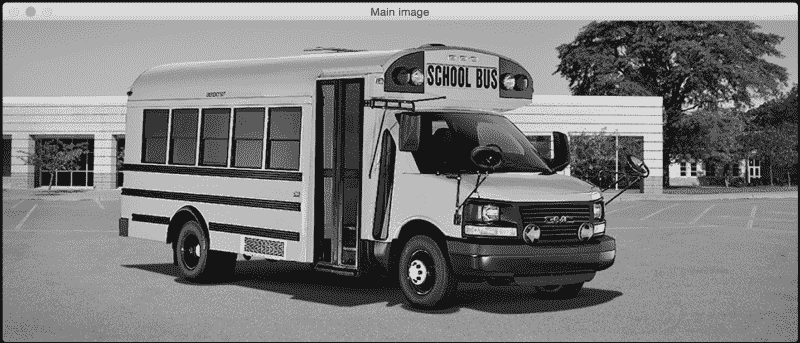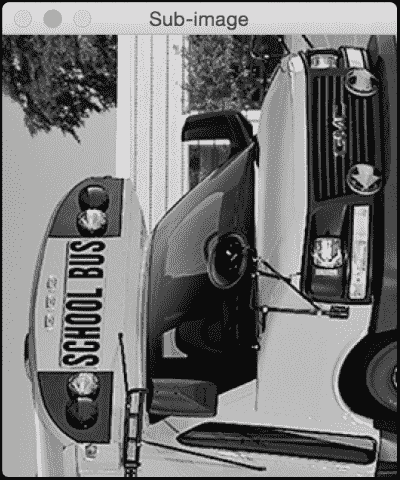import sys

import cv2
import numpy as np

def draw_matches(img1, keypoints1, img2, keypoints2, matches):
rows1, cols1 = img1.shape[:2]
rows2, cols2 = img2.shape[:2]

# Create a new output image that concatenates the two images together
output_img = np.zeros((max([rows1,rows2]), cols1+cols2, 3), dtype='uint8')
output_img[:rows1, :cols1, :] = np.dstack([img1, img1, img1])
output_img[:rows2, cols1:cols1+cols2, :] = np.dstack([img2, img2, img2])

# Draw connecting lines between matching keypoints
for match in matches:
# Get the matching keypoints for each of the images
img1_idx = match.queryIdx
img2_idx = match.trainIdx

(x1, y1) = keypoints1[img1_idx].pt
(x2, y2) = keypoints2[img2_idx].pt

# Draw a small circle at both co-ordinates and then draw a line
colour = (0,255,0)   # green
thickness = 1
cv2.line(output_img, (int(x1),int(y1)), (int(x2)+cols1,int(y2)), colour, thickness)

return output_img

if __name__=='__main__':
img1 = cv2.imread(sys.argv, 0)   # query image (rotated subregion)
img2 = cv2.imread(sys.argv, 0)   # train image (full image)

# Initialize ORB detector
orb = cv2.ORB()

# Extract keypoints and descriptors
keypoints1, descriptors1 = orb.detectAndCompute(img1, None)
keypoints2, descriptors2 = orb.detectAndCompute(img2, None)

# Create Brute Force matcher object
bf = cv2.BFMatcher(cv2.NORM_HAMMING, crossCheck=True)

# Match descriptors
matches = bf.match(descriptors1, descriptors2)

# Sort them in the order of their distance
matches = sorted(matches, key = lambda x:x.distance)

# Draw first 'n' matches
img3 = draw_matches(img1, keypoints1, img2, keypoints2, matches[:30])

cv2.imshow('Matched keypoints', img3)
cv2.waitKey()


## 我们如何匹配关键点？

bf = cv2.BFMatcher(cv2.NORM_HAMMING, crossCheck=True)


## 了解匹配器对象

matches = bf.match(descriptors1, descriptors2)


• item.distance：此属性为我们提供了描述符之间的距离。 距离越短表示匹配越好。
• item.trainIdx：此属性为我们提供了训练描述符列表中描述符的索引（在我们的情况下，它是完整图像中描述符的列表）。
• item.queryIdx：此属性为我们提供了查询描述符列表中描述符的索引（在我们的情况下，这是旋转子图像中的描述符列表）。
• item.imgIdx：此属性为我们提供训练图像的索引。

## 绘制匹配的关键点

output_img = np.zeros((max([rows1,rows2]), cols1+cols2, 3), dtype='uint8')


(x1, y1) = keypoints1[img1_idx].pt
(x2, y2) = keypoints2[img2_idx].pt


# 创建全景图像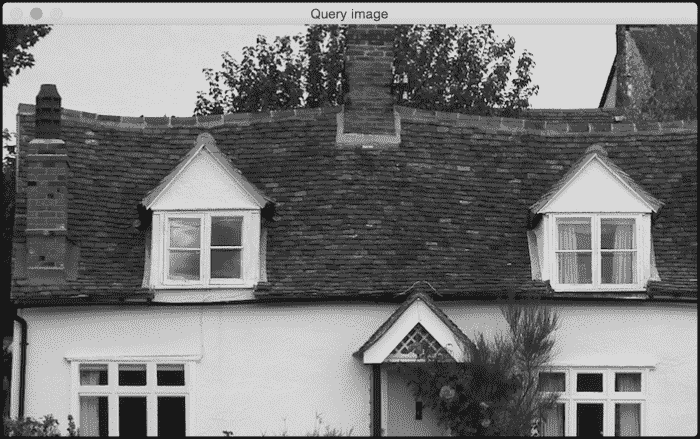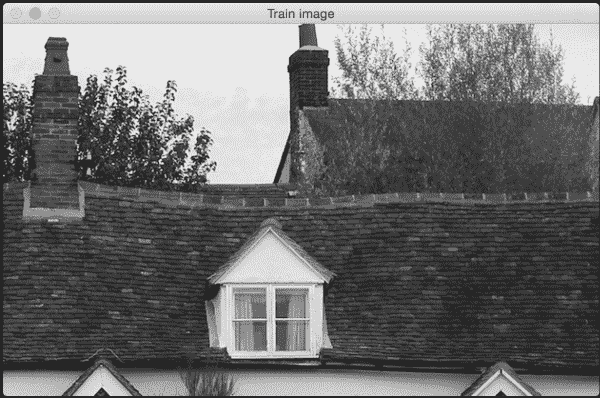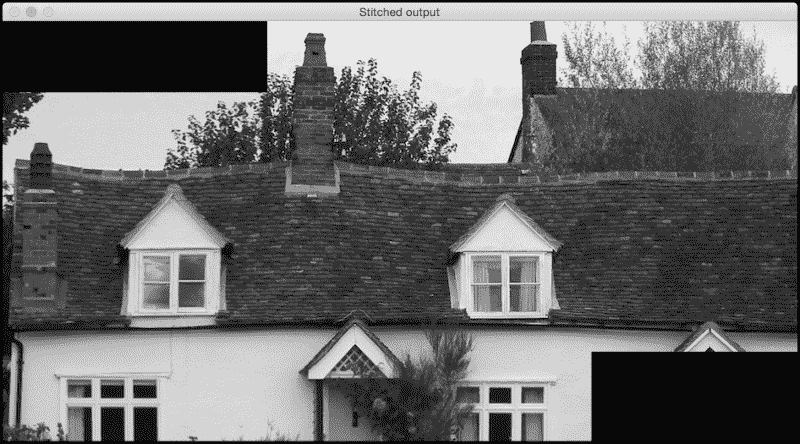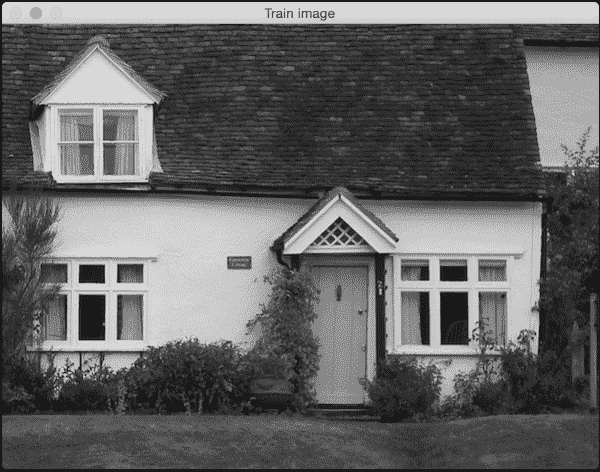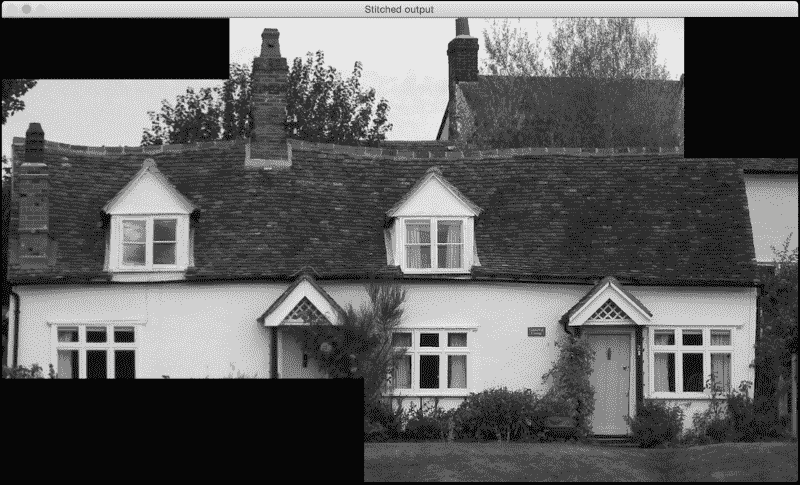import sys
import argparse

import cv2
import numpy as np

def argument_parser():
parser = argparse.ArgumentParser(description='Stitch two images together')
help="First image that needs to be stitched")
help="Second image that needs to be stitched")
required=False, default=10, help="Minimum number of matches required")
return parser

# Warp img2 to img1 using the homography matrix H
def warpImages(img1, img2, H):
rows1, cols1 = img1.shape[:2]
rows2, cols2 = img2.shape[:2]

list_of_points_1 = np.float32([[0,0], [0,rows1], [cols1,rows1], [cols1,0]]).reshape(-1,1,2)
temp_points = np.float32([[0,0], [0,rows2], [cols2,rows2], [cols2,0]]).reshape(-1,1,2)
list_of_points_2 = cv2.perspectiveTransform(temp_points, H)
list_of_points = np.concatenate((list_of_points_1, list_of_points_2), axis=0)

[x_min, y_min] = np.int32(list_of_points.min(axis=0).ravel() - 0.5)
[x_max, y_max] = np.int32(list_of_points.max(axis=0).ravel() + 0.5)
translation_dist = [-x_min,-y_min]
H_translation = np.array([[1, 0, translation_dist], [0, 1, translation_dist], [0,0,1]])

output_img = cv2.warpPerspective(img2, H_translation.dot(H), (x_max-x_min, y_max-y_min))
output_img[translation_dist:rows1+translation_dist, translation_dist:cols1+translation_dist] = img1

return output_img

if __name__=='__main__':
args = argument_parser().parse_args()
min_match_count = args.min_match_count

cv2.imshow('Query image', img1)
cv2.imshow('Train image', img2)

# Initialize the SIFT detector
sift = cv2.SIFT()

# Extract the keypoints and descriptors
keypoints1, descriptors1 = sift.detectAndCompute(img1, None)
keypoints2, descriptors2 = sift.detectAndCompute(img2, None)

# Initialize parameters for Flann based matcher
FLANN_INDEX_KDTREE = 0
index_params = dict(algorithm = FLANN_INDEX_KDTREE, trees = 5)
search_params = dict(checks = 50)

# Initialize the Flann based matcher object
flann = cv2.FlannBasedMatcher(index_params, search_params)

# Compute the matches
matches = flann.knnMatch(descriptors1, descriptors2, k=2)

# Store all the good matches as per Lowe's ratio test
good_matches = []
for m1,m2 in matches:
if m1.distance < 0.7*m2.distance:
good_matches.append(m1)

if len(good_matches) > min_match_count:
src_pts = np.float32([ keypoints1[good_match.queryIdx].pt for good_match in good_matches ]).reshape(-1,1,2)
dst_pts = np.float32([ keypoints2[good_match.trainIdx].pt for good_match in good_matches ]).reshape(-1,1,2)

M, mask = cv2.findHomography(src_pts, dst_pts, cv2.RANSAC, 5.0)
result = warpImages(img2, img1, M)
cv2.imshow('Stitched output', result)

cv2.waitKey()

else:
print "We don't have enough number of matches between the two images."
print "Found only %d matches. We need at least %d matches." % (len(good_matches), min_match_count)


# 如果图像彼此成角度怎么办？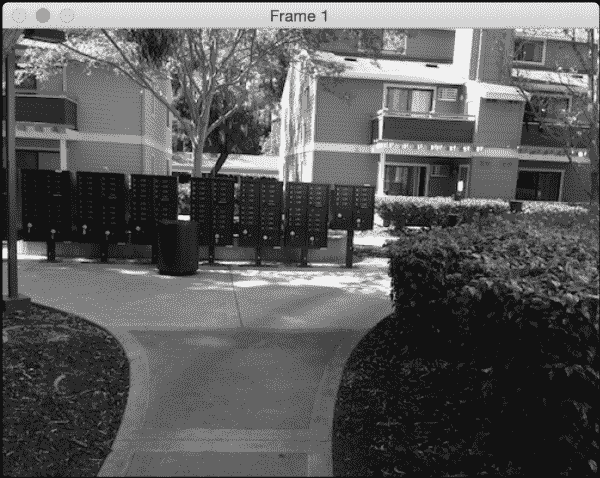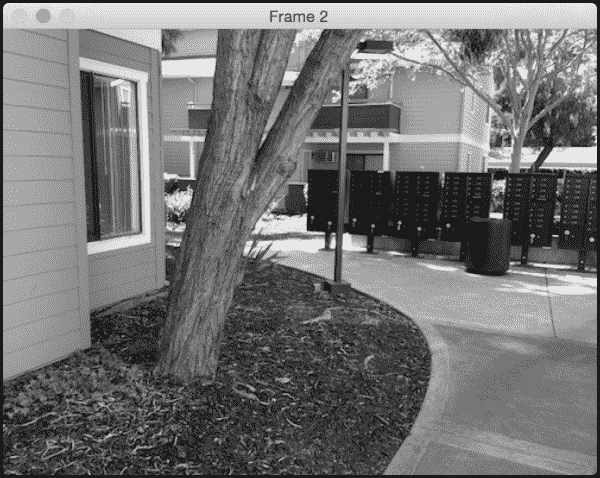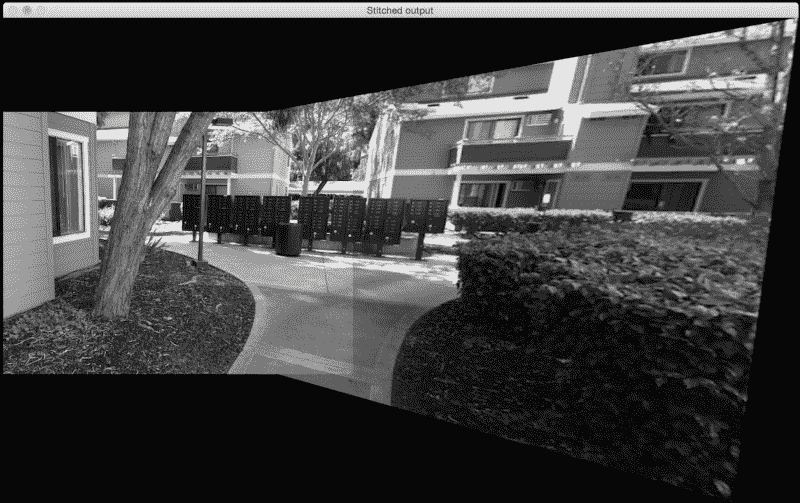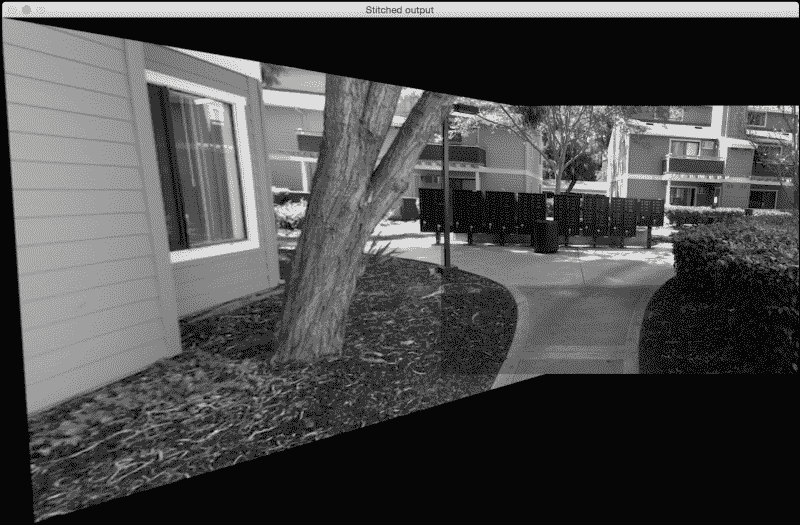## 为什么看起来很拉伸？

M, mask = cv2.findHomography(src_pts, dst_pts, cv2.RANSAC, 5.0)
result = warpImages(img2, img1, M)